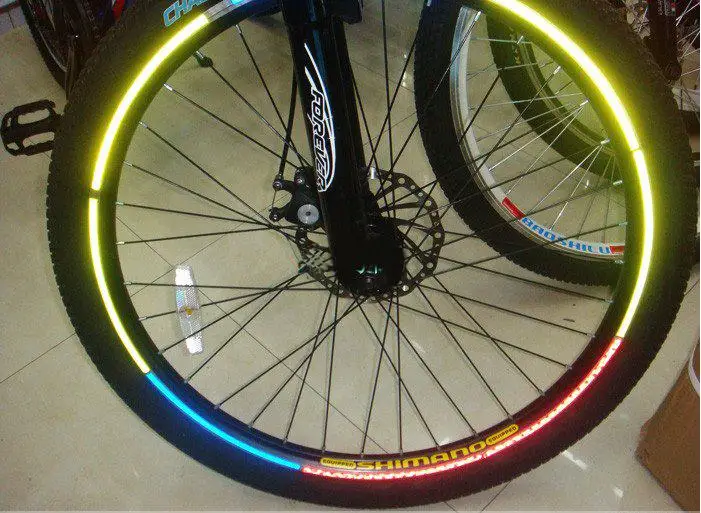# WTA

## Mrs Rigby’s Reasoning Question mistakes

On question 2 it says:”Circle the number closest to 500″

The numbers are 525 491 511 408 550. Miss Rigby wrote 511 but the closest one is 491, 511 is 11 off but 491 is only 9 off so 491 is the least away from 500.

On question 3 the question says “Calculate 735 + 2,669

Miss Rigby says it equals 10,019 but she put them in the wrong colunm  so it actually equals 3,444.

## Working out a maths question

If you struggle with maths , place value, then I am here to help you and explain a certain SATS style question. Sometimes teachers can help but most of the time ,what they say goes in one ear and straight out of the other. So if you are reading this post, then you are in luck because I am going to do a step by step guide on how to solve the question below:

A shop sells candles.      A plain candle which costs 35p each.     A star candle which costs 60p each.   And a stripe candle which costs 85p each.

Sapna buys 4 star candles and 2 stripe candles, how much does she pay altogether?                            So, first you read the question carefully. Then look at the writing that is in bold, which is altogether, and see what that word means. So the word altogether means you have to add in this certain problem.

Now you look at the question again and it says, ‘ Sapna buys 4 star candles.‘  So now you look at the star candles, and it says a star candle costs 60p each. Next you look at the 4 , because it says she buys 4 candles, and 60p , because that is how much one star candle costs, so now you times them together, which = 240p/ £2.40p.

So now you do the next step to the question, which is about the 2 stripe candles. Then you look at how much 1 stripe candle costs, which is 85p. Next you times 85p by 2 because Sapna bought 2 stripe candles. This = 170p/ £1.70p.

After that you add the costs of all the candles that Sapna bought, which was the 4 star candles which= £2.40p, and the 2 stripe candles which = £1.70. Afterwards you add them together which = £4.10p

Please comment if this helped you out. By KG

## What I Have Learned In Maths About Fractions Converting Into Decimals

Today in maths I found a pattern of converting all many ninths equals to the same numerator.If you had 2/9 in decimals it will be 0.22 that is the pattern what i have found it works on any one but when you get to wholes it is a bit different you see how many nines there are in the wholes and after the decimal point it will go back to the oringinal pattern Thank you for listening to this I will aprechiate all of this please comment down below

by Ayushma

## Different types of quadrilateral

This blog post will tell you all the facts of quadrilaterals .

This  is an really important fact that you need to know . Rectangles are shapes that a have lines that will never meet . For example a square has 2 sets of parallel line . The opposite lines go across in train track 1 set up and 1 sat across . Another shape is a kite the lines of this shape will never meet , just like a rectangle  and a rhombus. ✅

But some parallel line shapes have 1 set of parallel lines . One shape that we know is a trapezium and that has 1 set that will never meet that goes across . But the 2 lines  that go up will meet and then the trapezium will then form into a triangle .

Now I will tell you all the types of parallelograms. Squares , rectangles , rhombus , kite /diamond  , isosceles  trapezium and a normal trapezium . And that is all you need to know!

## What maths can you see?

Comment the maths that you can see in this picture:## emjio maths+= 100+= 75= -1

What does each one mean?

## 8 time tables and 13 time tables

1×8=82×8=16

3×8=24

4×8=32

5×8=40

6×8=48

7×8=56

8×8=64

9×8=72

10×8=80

11×8=88

12×8=96

13 time table

13×1=13

13×2=26

13×3=39

13×4=52

13×5=65

13×6=78

13×7=91

13×8=104

13×9=117

13×10=130

13×11=143

13×12=156

## Halloween AlgebraWhat can you tell me about the emojis? How much are they worth? Can you comment with your own statements?

## Maths Experts Partitioning

The maths experts have made a video for Year 2 about partitioning.